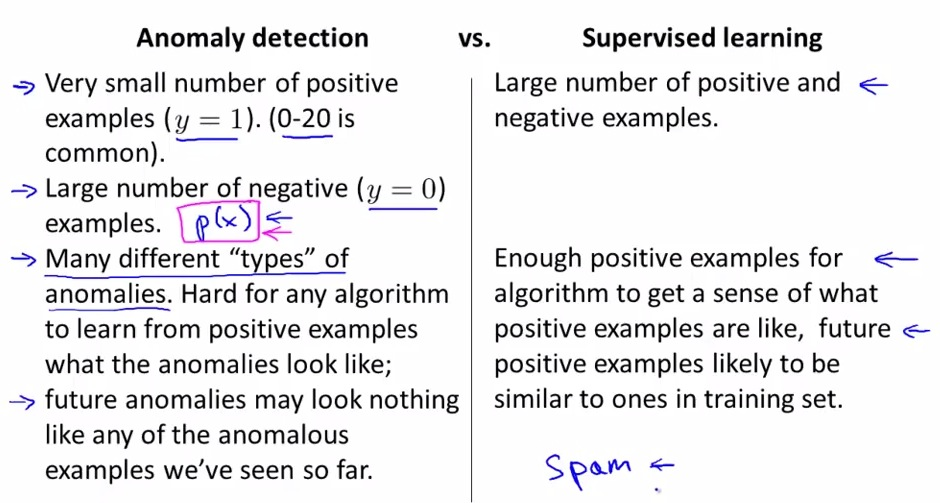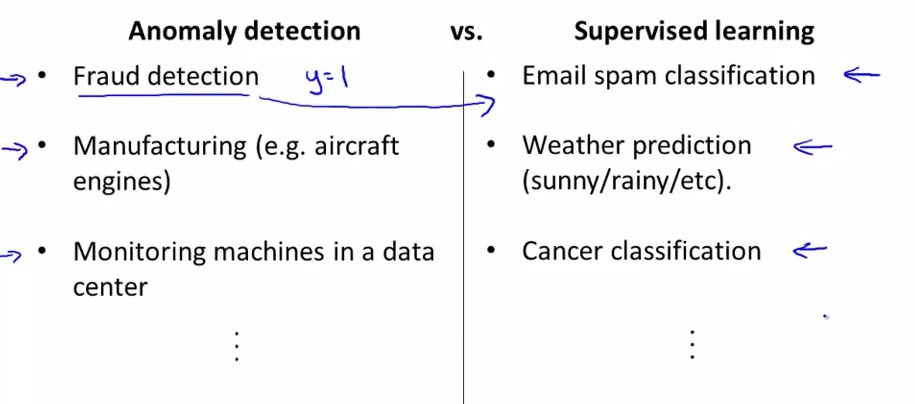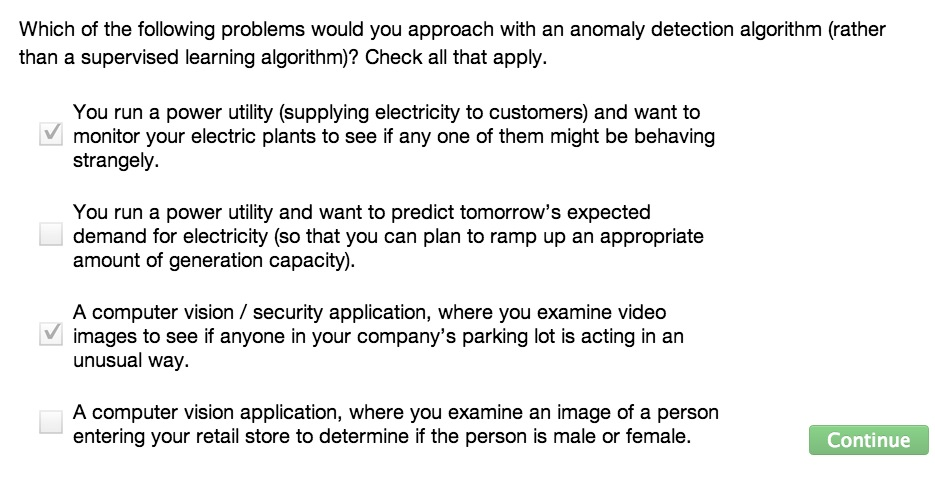• By now we already knew that we can evaluate Anomaly Detection by label the data whether is normal(y = 0) or anomalous(y = 1)
• Then question remain unanswered? When do we prefer to use Anomaly Detection over Supervised Learning?• So in Anomaly Detection we can choose it based on amount of positive and negative examples. The positive examples has very large examples (can override the negative examples itself).
• While in Supervised Learning, the negative and positive examples have a fair amount of data.
• In Anomaly Detection, even few negative examples, can still match the graph provided by the Gaussian Distribution. So we can leave the negative examples in training set, and offer the rest to cv and test set
• The spam examples is exceptional examples. Eventhough there's spam that relatively small, the spam category itself can give largerly different category. So for this particular examples, we use Supervised Learning• So for lot of negative/anomalous examples, or many variance involved, it is recommended to choose Supervised Learning
• If it doesn't (like aircraft engine examples) then we choose the other way around, Anomaly Detection• We learned for many specific problems from each category Anomaly Detection and Supervised Learning
• For other examples that we may yet see in the future, it is common tho choose Anomaly Detection